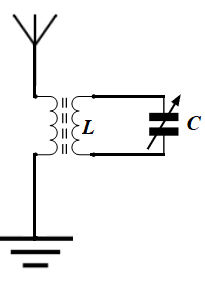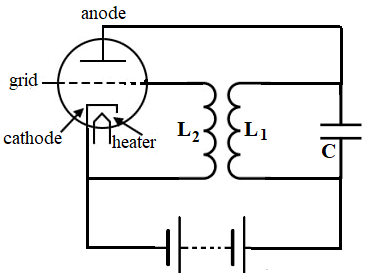Saturday, December 11, 2021

Notes to a video lecture on http://www.unizor.com

Radio transmission is a process of generating oscillations of electromagnetic field.
In this lecture we'll attempt to put together a simple generator, kind of those created in the beginning of the radio era.

In the previous lecture we discussed a simple LC circuit and connected to it a receiving antenna as a device that can capture the oscillations of electromagnetic field and induce the oscillations of electric current of a particular frequency in the circuit.This device accepts all electromagnetic waves of all frequencies into an antenna and, because of the resonance of a certain frequency with a circuit's own frequency, selects a particular frequency out of many received ones.

In theory, the same device can work in reverse - if there is an oscillating with certain frequency electric current in the circuit, it will induce the oscillations of an electric current in an antenna, which, in turn, will create electromagnetic waves around this antenna, oscillating with the same frequency.
If these electromagnetic waves are of certain strength, the device will serve as a transmitter.

To make this design work, we have to assure an uninterrupted oscillations of the current in our circuit with its own inherent frequency.
Generally speaking, the oscillations in our circuit are damped because of natural resistance. Let's design a system with some sort of energy support to compensate the damping effect of resistance in a circuit.

Consider a swing at the children's playground. After initial push it will eventually stop because of a friction, unless we give another push. That new push should be synchronized with a movement of a swing and directed along its movement. So, periodic push in some direction at every moment when a swing moves in that direction is a solution.

Let's design an electronic circuit that does similar supporting pushes to electric current.
One of many kinds of circuits that delivers this solution is below.
It utilizes a triode as an ON/OFF switch to turn ON or OFF the current from a battery that feeds a circuit exactly at the moments it's needed to support loss of energy due to circuit resistance.Assume, there are some oscillations in a circuit that contains inductor L1 and capacitor C.
These oscillations cause the variable electric current in the inductor L1, which, in turn, induces the electromotive force in the inductor L2.

Considering every circuit has certain resistance, the oscillations of electric current in the L1C circuit, if not connected to the inductor L2, would be damped and their amplitude would gradually diminish towards zero.

Note, however, that the oscillations of the electric current in the L1C circuit create a variable magnetic field with a variable magnetic flux going through the inductor L1 and, since it's paired with inductor L2, through L2 as well.

Since this magnetic flux is variable, it induces an electromotive force in inductor L2 proportional to a speed of change of the magnetic flux.
This variable electromotive force, in turn, causes a grid of a triode to be periodically charged positively or negatively.

When the grid is positive, electrons from the hot cathode go through a grid to the anode and feed the capacitor, thus compensating losses of energy due to resistance of a circuit.
If, however, the grid is negative, there is no current going from a cathode to an anode and capacitor is not receiving any charge from a battery.

To properly calculate the resulting oscillations of the current in the circuit we have to include this extra dosage of electricity supplied by a battery when the triode is open. This significantly complicates the calculations, and we will not go through this.
All we can state is that, with properly evaluated parameters of all components of this circuit, the feed from a battery will be exactly at the time the capacitor needs it, and the oscillations of the current in the circuit will be sustained as long as the battery has energy in it.

The above is just an idea. To practically implement it, we need to go through some calculations, which are beyond the scope of this course.

The above example is based on feeding a capacitor with additional charge. Alternatively, we can design a circuit, when a battery would feed an inductor with extra electromotive power.

Many other ideas and designs for transmitters were developed throughout the time, all have certain pluses and minuses, but the main principle of synchronously turn on the flow of new energy into an oscillator to compensate the losses due to resistance is still at the foundation of them all.